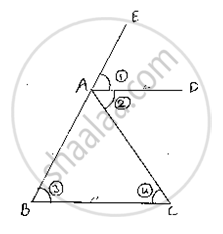# If the Bisector of the Exterior Vertical Angle of a Triangle Be Parallel to the Base. Show that the Triangle is Isosce - Mathematics

If the bisector of the exterior vertical angle of a triangle be parallel to the base. Show that the triangle is isosce

#### Solution

Given that the bisector of the exterior vertical angle of a triangle is parallel to the base and we have to prove that the triangle is isosceles

Let ABC be a triangle such that AD is the angular bisector of exterior vertical angle EAC and AD || BC

Let∠EAD = (1), ∠DAC = (2), ∠ABC = (3) and∠ACB = (4)

(1) = (2)        [   ∵ AD is bisector of , ∠EAC

(1)=(3)       [Corresponding angles]

and (2) = (4)    [alternative angle]

⇒ (3) = (4) ⇒AB = AC

Since, in ΔABC, two sides AB and AC are equal we can say that

DABC is isoscelesConcept: Concept of Triangles - Sides, Angles, Vertices, Interior and Exterior of Triangle
Is there an error in this question or solution?

#### APPEARS IN

RD Sharma Mathematics for Class 9
Chapter 12 Congruent Triangles
Exercise 12.3 | Q 2 | Page 47
Share